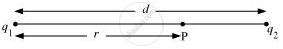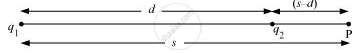# Two charges 5 × 10−8 C and −3 × 10−8 C are located 16 cm apart. At what point(s) on the line joining the two charges is the electric potential zero? Take the potential at infinity to be zero. - Physics

Numerical

Two charges 5 × 10−8 C and −3 × 10−8 C are located 16 cm apart. At what point(s) on the line joining the two charges is the electric potential zero? Take the potential at infinity to be zero.

#### Solution

There are two charges,

q1 = 5 × 10−8 C

q2 = −3 × 10−8 C

Distance between the two charges, d = 16 cm = 0.16 m

Consider a point P on the line joining the two charges, as shown in the given figure.r = Distance of point P from charge q1

Let the electric potential (V) at point P be zero.

Potential at point P is the sum of potentials caused by charges q1 and q2 respectively.

∴ "V" = q_1/(4piin_0"r") + "q"_2/(4piin_0("d" - "r")) ........(i)

Where,

in_0 = Permittivity of free space

For V = 0, equation (i) reduces to

"q"_1/(4piin_0"r") = -"q"_2/(4piin_0("d" - "r"))

"q"_1/"r" = -"q"_2/("d" - "r")

(5xx10^-8)/"r" = -(-3 xx 10^-8)/(0.16 - "r")

0.16/"r" = 8/5

∴ r = 0.1 m = 10 cm

Therefore, the potential is zero at a distance of 10 cm from the positive charge between the charges.

Suppose point P is outside the system of two charges at a distance s from the negative charge, where potential is zero, as shown in the following figure.For this arrangement, potential is given by,

"V" = "q"_1/(4piin_0"s") + "q"_2/(4 piin_0("s" -"d")) ......(ii)

For V = 0, equation (ii) reduces to

"q"_1/(4piin_0"s") = "q"_2/(4piin_0("s" - "d"))

"q"_1/"s" = -"q"_2/("s" - "d")

(5 xx 10^-8)/"s" = ((-3 xx 10^-8))/(("s" - 0.16))

1-0.16/"s" = 3/5

0.16/"s" = 2/5

∴ s = 0.4 m = 40 m

Therefore, the potential is zero at a distance of 40 cm from the positive charge outside the system of charges.

Concept: Potential Due to an Electric Dipole
Is there an error in this question or solution?

#### APPEARS IN

NCERT Physics Part 1 and 2 Class 12
Chapter 2 Electrostatic Potential and Capacitance
Exercise | Q 2.1 | Page 86
NCERT Class 12 Physics Textbook
Chapter 2 Electrostatic Potential and Capacitance
Exercise | Q 1 | Page 87
Share